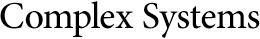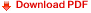## High-Probability Trajectories in the Phase Space and the System ComplexityDan C. Marinescu
Computer Science Division
Department of Electrical Engineering and Computer Science
University of Central Florida, Orlando, FL 32816, USA
dcm@cs.ucf.edu
www.cs.ucf.edu/~dcm/

#### Abstract

The dynamic behavior of a system can be modeled as the trajectory of the system in the phase space. A phase space is an abstraction where each possible state of the system is represented by a unique point; each dimension of the phase space represents a degree of freedom of the system. Individual trajectories have different probabilities, with some of them more likely than others. For a complex system, it is conjectured that the highly probable trajectories in the phase space are dominant. Random walks are analyzed for fully connected finite state machines. We show that the cardinality of the set of highly probable trajectories is very large; its lower bound is exponential in the number of states traversed by the random walk and in an expression of the entropy of a system.

https://doi.org/10.25088/ComplexSystems.22.3.233